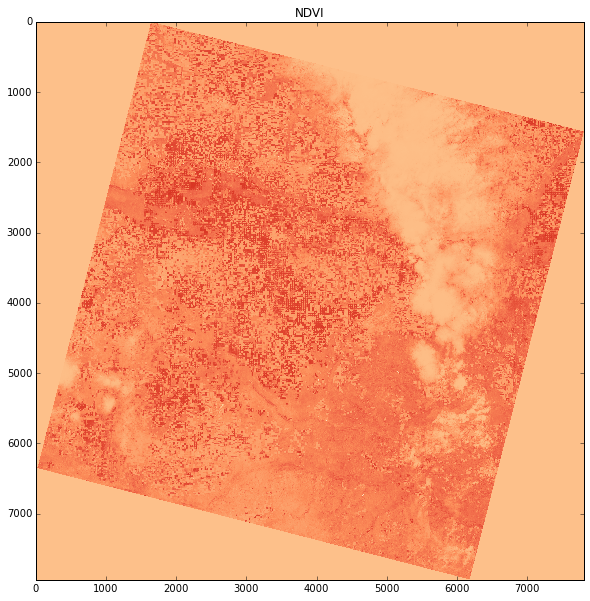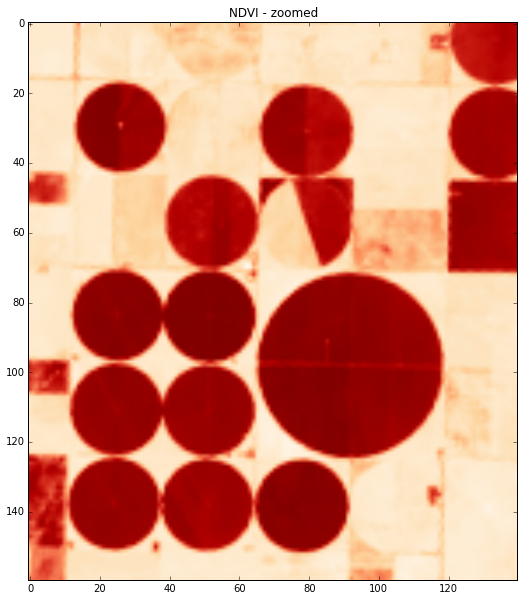# Normalized Difference Vegetation Index¶

This notebook is part of the collection accompanying the talk "Analyzing Satellite Images With Python Scientific Stack" by Milos Miljkovic given at PyData NYC 2014. The content is BSD licensed.

The normalized difference vegetation index (NDVI) is used to assess the state of vegetation. In living plants chlorophyll-A, from the photosynthetic machinery, strongly absorbs red color; on the other hand, near-infrared light is strongly reflected. Live, healthy vegetation reflects around 8% of red light and 50% of near-infrared light. Dead, unhealthy, or sparse vegetation reflects approximately 30% of red light and 40% of near-infrared light.

The NDVI is calculated as:

$$NDVI = \frac{\lambda_{NIR} - \lambda_{red}}{\lambda_{NIR} + \lambda_{red}}$$

where:

• $\lambda_{NIR}$ is near-infrared Band 5
• $\lambda_{red}$ is color red Band 4

By its formulation, NDVI ranges from -1 to +1. In practice, an area of an image containing living vegetation will have NDVI in the range 0.3 to 0.8. High water content clouds and snow will have negative values of the index. Bodies of water, having low reflectance in both Band 4 and 5, exhibit very low positive or negative index. Soil, having slightly higher reflectance in near-infrared than in red, will produce low positive values of the index.

Calculation of the NDVI is sensitive to to a few factors:

• Atmospheric composition and appropriate modeling influence the calculation, especially if the correct water and aerosol content are initially incorrectly estimated.
• Thin, hard to spot clouds like cirrus can significantly affect the calculation.
• Sensor effects such as Sun angle not being calculated on per-pixel basis can influence the index estimation.
In :
# To install watermark extension execute:
# %install_ext https://raw.githubusercontent.com/HyperionAnalytics/watermark/master/watermark.py

In :
%watermark -v -m -p numpy,scikit-image,matplotlib

CPython 3.4.2
IPython 2.3.1

numpy 1.9.1
scikit-image 0.10.1
matplotlib 1.4.2

compiler   : GCC 4.2.1 (Apple Inc. build 5577)
system     : OS X 10.10.1
release    : 14.0.0
machine    : x86_64
processor  : Intel(R) Core(TM) i7-3740QM CPU @ 2.70GHz
CPU cores  : 8
interpreter: 64bit

In :
import re
import numpy as np
from skimage import io, exposure
from matplotlib import pyplot as plt

In :
%matplotlib inline

In :
def read_band(n):
"""
Input:
n - integer in the range 1-11
Output:
img - 2D array of uint16 type
"""
if n in range(1, 12):
tif_list = !ls *.TIF
band_name = 'B' + str(n) + '.TIF'
img_idx = [idx for idx, band_string in enumerate(tif_list) if band_name in band_string]
return img
else:
print('Band number has to be in the range 1-11!')

In :
def image_show(img, color_map, title):
"""
Show image
Input:
img - 2D array of uint16 type
color_map - string
title - string
"""
fig = plt.figure(figsize=(10, 10))
fig.set_facecolor('white')
plt.imshow(img, cmap=color_map)
plt.title(title)
plt.show()

In :
def image_histogram(img):
"""
Plot image histogram
Input:
img - 2D array of uint16 type
"""
co, ce = exposure.histogram(img)

fig = plt.figure(figsize=(10, 7))
fig.set_facecolor('white')
plt.plot(ce[1::], co[1::])
plt.show()

In :
def image_adjust_brightness(img, limit_left, limit_right, color_map, title):
"""
Adjust image brightness and plot the image
Input:
img - 2D array of uint16 type
limit_left - integer
limit_right - integer
color_map - string
title - string
"""
img_ha = exposure.rescale_intensity(img, (limit_left, limit_right))

fig = plt.figure(figsize=(10, 10))
fig.set_facecolor('white')
plt.imshow(img_ha, cmap=color_map)
plt.title(title)
plt.show()

return img_ha

In :
def get_gain_bias_angle(n):
"""
Get band reflectance gain, bias,
and Sun elevation angle
Input:
n - integer in the range 1-11
Output:
gain - float
bias - float
"""
if n in range(1, 10):
n_str = str(n)
s_g = 'REFLECTANCE_MULT_BAND_' + n_str + ' = '
s_b = 'REFLECTANCE_ADD_BAND_' + n_str + ' = '

fn = !ls *_MTL.txt

f = open(fn, 'r+')

search_str_g = '(?<=' + s_g + ').*'
search_str_b = '(?<=' + s_b + ').*'
search_str_a = '(?<=' + 'SUN_ELEVATION = ' + ').*'

for line in f:
s0 = re.search(search_str_a, line)
s1 = re.search(search_str_g, line)
s2 = re.search(search_str_b, line)
if s0:
angle = float(s0.group(0))
elif s1:
gain = float(s1.group(0))
elif s2:
bias = float(s2.group(0))

f.close()

return gain, bias, angle
else:
print('Band number has to be in the range 1-9!')


### Load Band 4 and 5 images from dataset LC80300342014219LGN00¶

In :
cd /Users/kronos/gis/l8/kansas_aug/

/Users/kronos/gis/l8/kansas_aug

In :
b4 = read_band(4)


### Get reflectance gain and bias, and Sun elevation angle; calculate top-of-atmosphere reflectance¶

In :
b4_gain, b4_bias, angle = get_gain_bias_angle(4)
b5_gain, b5_bias, angle = get_gain_bias_angle(5)

b4_lambda_refl  = (b4_gain * b4 + b4_bias) / np.sin(angle)
b5_lambda_refl  = (b5_gain * b5 + b5_bias) / np.sin(angle)


### Calculate NDVI¶

In :
ndvi = (b5_lambda_refl - b4_lambda_refl) / (b5_lambda_refl + b4_lambda_refl)

In :
img_ha = image_adjust_brightness(ndvi, -0.7695, 1.459, 'OrRd', 'NDVI')### Central pivot irrigation system¶

Each of the smaller circular crop fields is a half a mile in diameter. The largest crop field is one mile in diameter.

In :
image_show(ndvi[3440:3600, 3060:3200], 'OrRd', 'NDVI - zoomed')